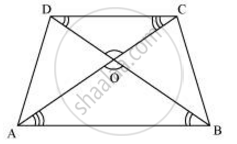Share

# Diagonals of a trapezium ABCD with AB || DC intersect each other at the point O. If AB = 2CD, find the ratio of the areas of triangles AOB and COD. - CBSE Class 10 - Mathematics

#### Question

Diagonals of a trapezium ABCD with AB || DC intersect each other at the point O. If AB = 2CD, find the ratio of the areas of triangles AOB and COD.

#### SolutionSince AB || CD,

∴ ∠OAB = ∠OCD and ∠OBA = ∠ODC (Alternate interior angles)

In ΔAOB and ΔCOD,

∠AOB = ∠COD (Vertically opposite angles)

∠OAB = ∠OCD (Alternate interior angles)

∠OBA = ∠ODC (Alternate interior angles)

∴ ΔAOB ∼ ΔCOD (By AAA similarity criterion)

:.(ar(ΔAOB))/(ar(COD)) = ((AB)/(CD))^2

Since AB = 2 CD

:. (ar(triangleAOB))/(ar(triangleCOD)) =((2CD)/(CD))^2 =4/1=4:1

Is there an error in this question or solution?

#### APPEARS IN

NCERT Solution for Mathematics Textbook for Class 10 (2019 to Current)
Chapter 6: Triangles
Ex. 6.40 | Q: 2 | Page no. 143

#### Video TutorialsVIEW ALL 

Solution Diagonals of a trapezium ABCD with AB || DC intersect each other at the point O. If AB = 2CD, find the ratio of the areas of triangles AOB and COD. Concept: Areas of Similar Triangles.
S